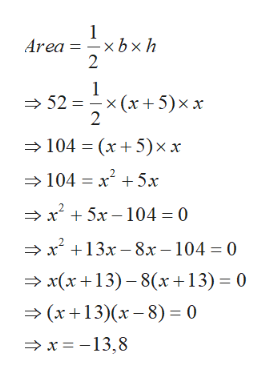# The area of a triangular plot of land is 52 yds2 .  The base is 5 yds longer than the height.   Find the base and the height.

Question
12 views

The area of a triangular plot of land is 52 yds.  The base is 5 yds longer than the height.   Find the base and the height.

check_circle

star
star
star
star
star
1 Rating
Step 1

Given that

Area of triangular plot of land = 52 yds2

Assume that height of triangular plot = x

So base of triangular plot = x+5

Step 2

Area of triangular plot = base*height/2help_outlineImage Transcriptionclose1 Area = xb x h 2 1 x (x 5)xx 2 52 104 (x5) xx 104 x 5x -x +5x-104= 0 x13x-8x-104 = 0 x(x13)-8(x+13) 0 (x13)(x-8) 0 x =-13,8 fullscreen
Step 3

Since x=-13 is not possible as height cannot be negative, so heigh...

### Want to see the full answer?

See Solution

#### Want to see this answer and more?

Solutions are written by subject experts who are available 24/7. Questions are typically answered within 1 hour.*

See Solution
*Response times may vary by subject and question.
Tagged in

### Applications of Mathematics